• 宽度优先搜索和广度优先搜索
2022-05-30 18:29:42

## 算法-宽度优先搜索

一、宽度优先搜索

广度优先或横向优先搜索，是一种图形搜索算法。简单的说，BFS是从根节点开始，沿着树的宽度遍历树的节点。如果所有节点均被访问，则算法中止。

DFS（Depth-First-Search）深度优先搜索：对每一个可能的分支路径深入到不能再深入为止，而且每个结点只能访问一次。要特别注意的是，二叉树的深度优先遍历比较特殊，可以细分为先序遍历、中序遍历、后序遍历。（它的目的是要达到被搜索结构的叶结点 ）

BFS： 广度优先搜索又叫层次遍历，从上往下对每一层依次访问，在每一层中，从左往右（也可以从右往左）访问结点，访问完一层就进入下一层，直到没有结点可以访问为止。

深度优先搜索和宽度优先搜索区别

• 二叉树的深度优先遍历的非递归的通用做法是采用栈，广度优先遍历的非递归的通用做法是采用队列
• 深度优先搜素算法：不全部保留结点，占用空间少；有回溯操作(即有入栈、出栈操作)，运行速度慢。
• 广度优先搜索算法：保留全部结点，占用空间大； 无回溯操作(即无入栈、出栈操作)，运行速度快。

二、小岛问题

class Solution {
public int numIslands(char[][] grid) {
if(grid == null || grid.length == 0) {
return 0;
}
if (grid == null || grid.length == 0) {
return 0;
}
int row = grid.length;
int column = grid.length;
boolean[][] visited = new boolean[row][column];
int number = 0;
for (int i = 0; i < row; i++) {
for (int j = 0; j < column; j++) {
if (grid[i][j] == '1' && !visited[i][j]) {
bfs(grid, i, j, visited);
number++;
}
}
}
return number;
}

public void bfs(char[][] grid, int i, int j, boolean[][] visited) {
int[] kx ={1, -1, 0, 0};
int[] ky ={0, 0, 1, -1};
visited[i][j] = true;
Queue<Integer> xQueue = new LinkedList<>();
Queue<Integer> yQueue = new LinkedList<>();
xQueue.offer(i);
yQueue.offer(j);
while (!xQueue.isEmpty()) {
int currentX = xQueue.poll();
int currentY = yQueue.poll();
for (int k = 0; k < 4; k++) {
int newX = currentX + kx[k];
int newY = currentY + ky[k];
if (newX >= 0 && newY >= 0 && newX < grid.length && newY < grid.length && !visited[newX][newY]) {
if (grid[newX][newY] == '1') {
xQueue.offer(newX);
yQueue.offer(newY);
visited[newX][newY] = true;
}
}
}
}
}
}


三、单词梯问题

public class Solution {
/*
* @param start: a string
* @param end: a string
* @param dict: a set of string
* @return: An integer
*/
public int ladderLength(String start, String end, Set<String> dict) {
// write your code here
int steps = 1;
if (dict == null) {
return 0;
}
Queue<String> queue = new LinkedList<>();
queue.offer(start);
Set<String> duplicate = new HashSet<>();
while (!queue.isEmpty()) {
int size = queue.size();
steps++;
for (int i = 0; i < size; i++) {
String word = queue.poll();
List<String> nextWords = getNext(word, dict);
for (String next: nextWords) {
if (duplicate.contains(next)) {
continue;
}
if (next.equals(end)) {
return steps;
}
queue.offer(next);
}
}
}
return -1;
}

public List<String> getNext(String word, Set<String> dict) {
List<String> next = new ArrayList<>();
for (char i = 'a'; i <= 'z'; i++) {
for (int j = 0; j < word.length(); j++) {
String potentialNext = changedWord(word, i, j);
if (dict.contains(potentialNext)) {
}
}
}
return next;
}

public String changedWord(String word, char c, int i) {
char[] words = word.toCharArray();
words[i] = c;
return new String(words);
}

}leetcode 广度优先 宽度优先搜索
更多相关内容
• 本源码是针对八数码问题的C语言实现方法，有较详细的注释。着重于广度搜索条件。大概就是这样吧。。。为啥这资源描述要这么多字。。。。
• 初始状态目标状态可自定采用宽度优先搜索算法，编程实现八数码问题的求解。初始状态目标状态可自定采用宽度优先搜索算法，编程实现八数码问题的求解。初始状态目标状态可自定采用宽度优先搜索算法，编程实现八...
• 广度优先搜索 - 宽度优先搜索 - 横向优先搜索 (breadth-first search，BFS) 1. 广度优先搜索 - 宽度优先搜索 - 横向优先搜索 (breadth-first search，BFS) depth-first search，DFS：深度优先搜索 breadth-first ...

# 广度优先搜索 - 宽度优先搜索 - 横向优先搜索 (breadth-first search，BFS)

## 1. 广度优先搜索 - 宽度优先搜索 - 横向优先搜索 (breadth-first search，BFS)

depth-first search，DFS：深度优先搜索


Breadth-first search (BFS) is an algorithm for traversing or searching tree or graph data structures. It starts at the tree root (or some arbitrary node of a graph, sometimes referred to as a search key), and explores all of the neighbor nodes at the present depth prior to moving on to the nodes at the next depth level.
广度优先搜索 (BFS) 是用于遍历或搜索树或图形数据结构的算法。它从树的根部 (或图的某个任意节点，有时称为搜索关键字) 开始，并在移至下一个深度级别的节点之前先探索当前深度的所有邻居节点。

广度优先搜索 - 宽度优先搜索 - 横向优先搜索 (breadth-first search，BFS) 是一种图形搜索算法。BFS 是从根节点开始，沿着树的宽度遍历树的节点。如果所有节点均被访问，则算法中止。广度优先搜索的实现一般采用 open-closed 表。

It uses the opposite strategy as depth-first search, which instead explores the node branch as far as possible before being forced to backtrack and expand other nodes.
它使用与深度优先搜索相反的策略，而是在被迫回溯和扩展其他节点之前，尽可能地探索节点分支。以德国城市为示例的地图。城市间有数条道路相连接。(An example map of Southern Germany with some connections between cities.)从法兰克福开始运行广度优先搜索算法，所产生的广度优先搜索算法树。(The breadth-first tree obtained when running BFS on the given map and starting in Frankfurt.)广度优先搜索算法的动画示例 (Animated example of a breadth-first search) - 达到覆盖面积的作用

BFS 是一种盲目搜索法，目的是系统地展开并检查图中的所有节点，以找寻结果。它并不考虑结果的可能地址，彻底地搜索整张图，直到找到结果为止。BFS 并不使用经验法则算法。

从算法的观点，所有因为展开节点而得到的子节点都会被加进一个先进先出的队列中。一般的实现里，其邻居节点尚未被检验过的节点会被放置在一个被称为 open 的容器中 (例如队列或链表)，而被检验过的节点则被放置在被称为 closed 的容器中。(open-closed 表)

animate [ˈænɪmeɪt]：vt. 使有生气，使活泼，鼓舞，推动 adj. 有生命的


广度优先搜索 (BFS) 是图的一种遍历方式，它以广度优先进行搜索。简言之就是先访问图的顶点，然后广度优先访问其邻接点，然后再依次进行被访问点的邻接点，一层一层访问，直至访问完所有点，遍历结束。

### 1.1 无向图的广度优先搜索过程

遍历结果：A -> C -> D -> F -> B -> G -> E### 1.2 有向图的广度优先搜索

遍历结果：A -> B -> C -> E -> F -> D -> G## 2. 深度优先搜索 - 广度优先搜索

• 深度优先搜索占内存少但速度较慢，广度优先搜索占内存多但速度较快。
• 深度优先搜索与广度优先搜索的控制结构和产生系统很相似，唯一的区别在于对扩展节点选取上。
• 深度优先搜索与广度优先搜索两种算法每次都扩展一个节点的所有子节点，不同的是，深度优先下一次扩展的是本次扩展出来的子节点中的一个，而广度优先扩展的则是本次扩展的节点的兄弟点。在具体实现上为了提高效率，所以采用了不同的数据结构。

### 2.1 深度优先搜索 (depth-first search，DFS)

深度优先搜索用栈 (stack) 来实现，整个过程可以看做一个倒立的树形。

1. 把根节点压入栈中。
2. 每次从栈中弹出一个元素，搜索所有在它下一级的元素，把这些元素压入栈中。并把这个元素记为它下一级元素的前驱。
3. 找到所要找的元素时结束程序。
4. 如果遍历整个树还没有找到，结束程序。

### 2.2 广度优先搜索 - 宽度优先搜索 - 横向优先搜索 (breadth-first search，BFS)

广度优先搜索使用队列 (queue) 来实现，整个过程可以看做一个倒立的树形。

1. 把根节点放到队列的末尾。
2. 每次从队列的头部取出一个元素，查看这个元素所有的下一级元素，把它们放到队列的末尾。并把这个元素记为它下一级元素的前驱。
3. 找到所要找的元素时结束程序。
4. 如果遍历整个树还没有找到，结束程序。

## 3. 空间复杂度

说法一
所有节点都必须被存储，BFS 的空间复杂度为 O ( ∣ V ∣ + ∣ E ∣ ) O(|V|+|E|) ，其中 ∣ V ∣ |V| 是节点的数目，而 ∣ E ∣ |E| 是图中边的数目。

说法二
BFS 的空间复杂度为 O ( B M ) O(B^M) ，其中 B B 是最大分支系数，而 M M 是树的最长路径长度。由于对空间的大量需求，BFS 并不适合解非常大的问题。对于类似的问题，应用 IDDFS 以达节省空间的效果。

## 4. 时间复杂度

最差情形下，BFS 必须查找所有到可能节点的所有路径，因此其时间复杂度为 O ( ∣ V ∣ + ∣ E ∣ ) O(|V|+|E|) ，其中 ∣ V ∣ |V| 是节点的数目，而 ∣ E ∣ |E| 是图中边的数目。

## 5. 完全性

广度优先搜索算法具有完全性。无论图形的种类如何，只要目标存在，则 BFS 一定会找到。若目标不存在，且图为无限大，则 BFS 将不收敛 (不会结束)。

## 6. 最佳解

若所有边的长度相等，广度优先搜索算法是最佳解 - 亦即它找到的第一个解，距离根节点的边数目一定最少。但对一般的图来说，BFS 并不一定回传最佳解。这是因为当图形为加权图 (亦即各边长度不同) 时，BFS 仍然回传从根节点开始，经过边数目最少的解。而这个解距离根节点的距离不一定最短。这个问题可以使用考虑各边权值，BFS 的改良算法成本一致搜索法来解决。若非加权图形，则所有边的长度相等，BFS 就能找到最近的最佳解。

## 7. 广度优先搜索算法的应用

广度优先搜索算法能用来解决图论中的许多问题，例如：

• 查找图中所有连接组件 (Connected Component)。一个连接组件是图中的最大相连子图。
• 查找连接组件中的所有节点。
• 查找非加权图中任两点的最短路径。
• 测试一图是否为二分图。
• (Reverse) Cuthill-McKee 算法
reverse [rɪˈvɜːs]：v. 颠倒，撤销，反转，交换，放弃立场，倒车，打对方付费的电话，(使铅字、图案) 印成白或浅色 n. 逆向，相反，背面，倒档，失败，(美橄) 横式传球，(翻开书的) 左手页 adj. 相反的，背面的，颠倒的，反身的，(地层) 逆断的
activator ['æktɪveɪtə]：n. 催化剂，活化剂，激活剂，触媒剂


### 7.1 查找连接组件

由起点开始，运行广度优先搜索算法后所经过的所有节点，即为包含起点的一个连接组件。

## References

展开全文广度优先搜索 宽度优先搜索 BFS
• 广度宽度优先搜索，将八数码问题简化成三数码问题求解
• 宽度优先搜索（BFS）与双向广搜

百度百科的官方解释：宽度优先搜索算法（又称广度优先搜索）是最简便的图的搜索算法之一，这一算法也是很多重要的图的算法的原型。Dijkstra单源最短路径算法和Prim最小生成树算法都采用了和宽度优先搜索类似的思想。其别名又叫BFS，属于一种盲目搜寻法，目的是系统地展开并检查图中的所有节点，以找寻结果。换句话说，它并不考虑结果的可能位置，彻底地搜索整张图，直到找到结果为止。

这是一个很难硬说就能理解的知识点，但是理解了就会觉得很简单（事实上所有知识点都是这样），配图理解BFS。

假设有这么一个图，1是起点，5是终点。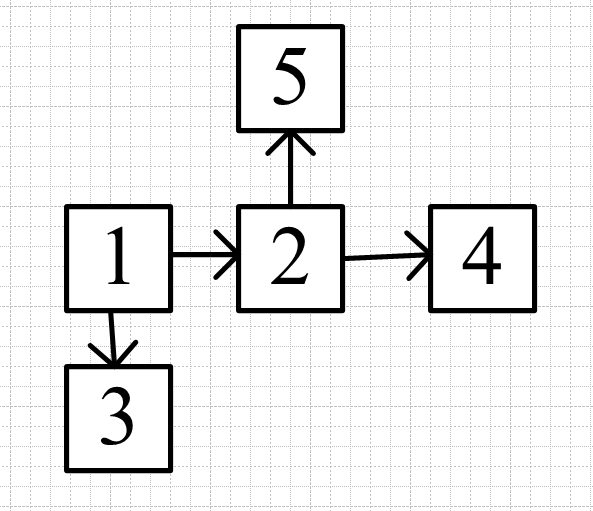遍历时将1首先加入队列（队列就是模拟BFS用的，栈是模拟DFS用的，应用的是队列先进先出和栈先进后出的特性）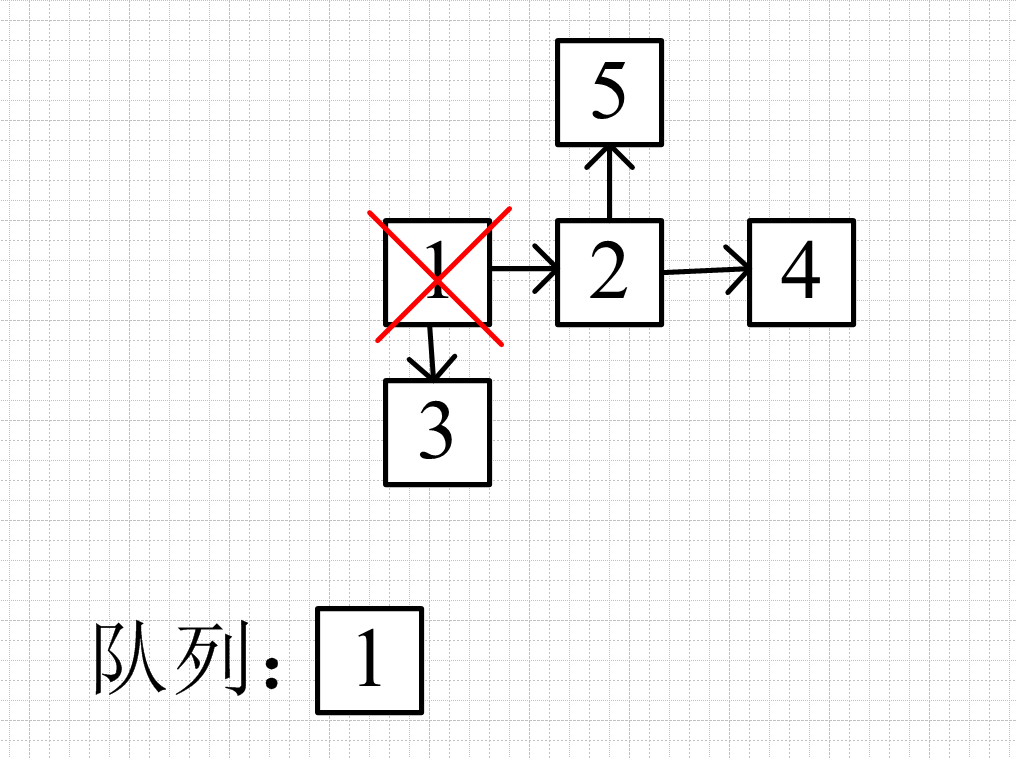然后应用一个循环模板

while(队列不为空){
弹出队头
遍历队头接下来的节点
将满足的节点加入队列
}

模拟while过程，将1弹出队列，遍历1相连的节点，将满足条件的加入队列（这里不设条件）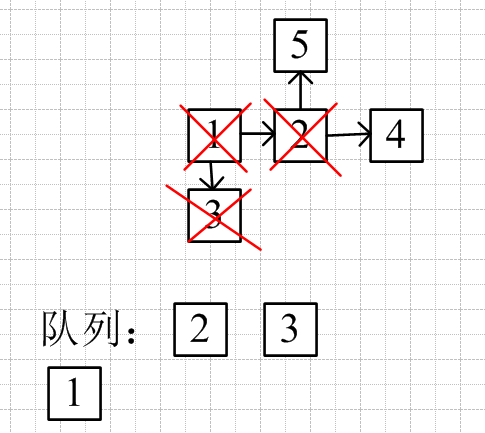弹出队头节点3，遍历与3相连的节点，与3连接的节点进队列（这里没有与3相连的），一直是从2的左边进入（即队尾进入），所以无论如何下一次都是先遍历与2相连的节点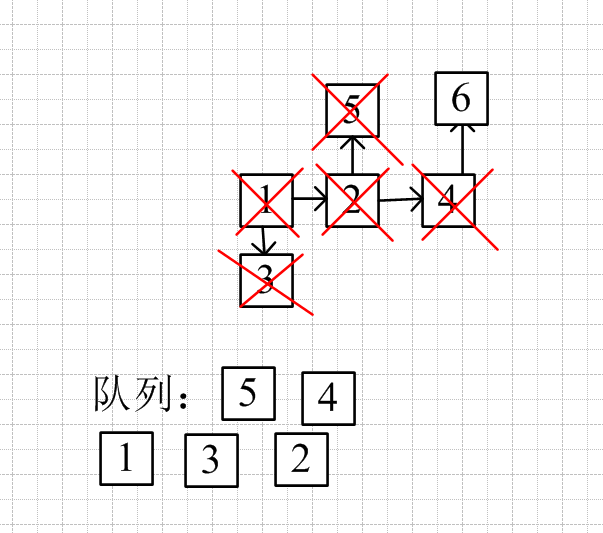比如在节点4后有一个相连的6，弹出4，加入6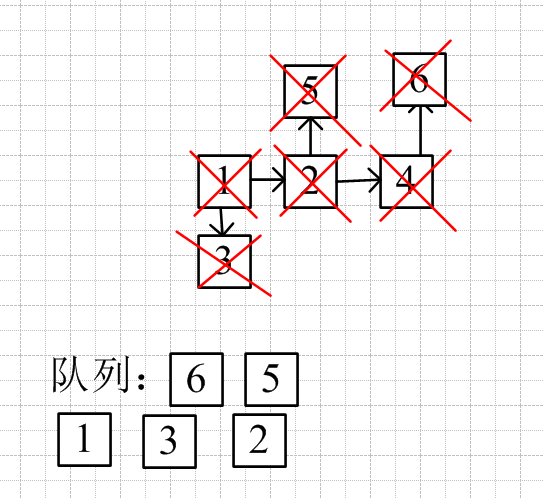但下一次的队头就是5了，而不是加入的6

这就是bfs，所有起点同步进行（类似同心圆向外扩散）

这里就会出现一个问题：如果每一个入队列的节点遍历之后都能带来接下来四个满足条件的节点，那么每一次遍历，队列就会扩大四倍，有一个比较简单的方法是引用双向广搜

双向广搜：起点终点同时加入队列，两边同时遍历，当两个节点的下一步有相交的节点，就是最短路径或者最短时间。

优化的部分可以看此图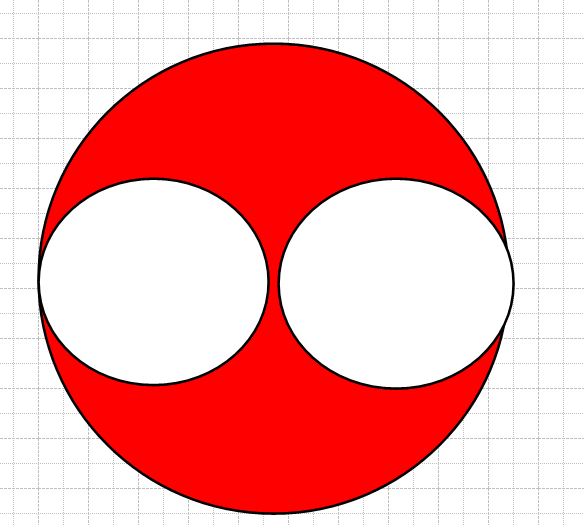红色部分是单向搜索比双向搜索多出来的部分，优化空间很大，同时也优化了时间

代码模板可变性很高，下面的看个乐就行了（毕竟bfs还是用处挺广泛的）

如果用queue模板的话记住front才是队头，如果遍历是用back就错了，因为pop弹出的front

#include "iostream"
#include "queue"
using namespace std;

struct Node{
int x;
int y;
}startNode,endNode;

int main(){
queue<Node>bfs;
bfs.push(startNode);
while(!bfs.empty()){
Node tempNode=bfs.front();
int x=tempNode.x;
int y=tempNode.y;
bfs.pop();

Node nextNode;
if(check(x+1,y)){//check是当这个条件成立时的随意一个函数，如果是迷宫
nextNode.x=x+1;//只要x+1,y这个位置不是墙壁就加入队列
nextNode.y=y;
bfs.push(nextNode);
}
if(check(x-1,y)){
nextNode.x=x-1;
nextNode.y=y;
bfs.push(nextNode);
}
if(check(x,y-1)){
nextNode.x=x;
nextNode.y=y-1;
bfs.push(nextNode);
}
if(check(x,y+1)){
nextNode.x=x;
nextNode.y=y+1;
bfs.push(nextNode);
}
}
}

展开全文数据结构 图搜索算法
• 广度优先搜索 / 宽度优先搜索 (Breadth First Search，BFS) - 层层递进 深度优先搜索方法可用于解决从迷宫起点开始寻找一条通往迷宫中目标位置最短路径的问题。广度优先搜索 / 宽度优先搜索 (Breadth First Search，...

# 广度优先搜索 / 宽度优先搜索 (Breadth First Search，BFS) - 层层递进

深度优先搜索方法可用于解决从迷宫起点开始寻找一条通往迷宫中目标位置最短路径的问题。广度优先搜索 / 宽度优先搜索 (Breadth First Search，BFS) 也可以解决这个问题。

使用二维数组来存储这个迷宫。最开始的时候在迷宫 (1, 1) 处，可以往右走或者往下走。深度优先搜索的方法是先往右边走，然后一直尝试下去，直到走不通的时候再回到这里。深度优先可以通过函数的递归实现。广度优先搜索 / 宽度优先搜索 (Breadth First Search，BFS) 方法通过一层一层扩展的方法来寻找目标位置。扩展时每发现一个点就将这个点加入到队列中，直至走到目标位置 (ph, qw) 时为止。

最开始在入口 (1, 1) 处，一步之内可以到达的点有 (1, 2)(2, 1)但是目标位置并不在这两个点上，只能通过 (1, 2)(2, 1) 这两点继续往下走。比如现在走到了 (1, 2) 这个点，之后又能够到达 (2, 2)。再看看通过 (2, 1) 又可以到达 (2, 2)(3, 1)。此时会发现 (2, 2) 这个点既可以从 (1, 2) 到达，也可以从 (2, 1) 到达，并且都只使用了 2 步。为了防止一个点多次被走到，这里需要一个数组来记录一个点是否已经被走到过。此时 2 步可以走到的点就全部走到了，有 (2, 2)(3, 1)，可是目标位置并不在这两个点上。看来没有别的办法，还得继续往下尝试，看看通过 (2, 2)(3, 1) 这两个点还能到达哪些新的没有走到过的点。通过 (2, 2) 这个点我们可以到达 (2, 3)(3, 2)，通过 (3, 1) 可以到达 (3, 2)(4, 1)。现在 3 步可以到达的点有 (2, 3)(3, 2)(4, 1)，依旧没有到达目标的所在点，所以我们需要继续重复刚才的做法，直到找到目标位置所在点为止。回顾一下刚才的算法，可以用一个队列来模拟这个过程。在这里我们用一个结构体数组来实现队列。

struct note {
int xw; // 横坐标
int yh; // 纵坐标
int step; // 步数
};

struct note que; // 因为地图大小不超过 50*50，因此队列扩展不会超过 2500
int map = { 0 }; // 用来存储地图
int book = { 0 }; // 数组 book 的作用是记录哪些点已经在队列中了，防止点被重复扩展，并全部初始化为 0

/* 最开始的时候需要进行队列初始化，即将队列设置为空 */
tail = 0;

// 第一步将 (1, 1) 加入队列，并标记 (1, 1) 已经走过。
que[tail].xw = 1;
que[tail].yh = 1;
que[tail].step = 0;
tail++;

book = 1;然后从 (1, 1) 开始，先尝试往右走到达了 (1, 2)

txw = que[head].xw + 1;


需要判断 (1, 2) 是否越界。

if ((txw < 1) || (txw > W) || (tyh < 1) || (tyh > H))
{
continue;
}


再判断 (1, 2) 是否为障碍物或者已经在路径中。

if ((0 == map[tyh][txw]) && (0 == book[tx][ty]))
{
}


如果满足上面的条件，则将 (1, 2) 入队，并标记该点已经走过。

book[tyh][txw] = 1; // bfs 每个点通常情况下只入队一次，同深搜不同，不需要将 book 数组还原
// 插入新的点到队列中
que[tail].xw = txw;
que[tail].yh = tyh;
que[tail].step = que[head].step + 1; // 步数是父亲的步数 + 1
tail++;接下来还要继续尝试往其他方向走。在这里我们规定一个顺序，即按照顺时针的方向来尝试 (右 -> 下 -> 左 -> 上)。我们发现从 (1, 1) 还是可以到达 (2, 1)，因此也需要将 (2, 1) 也加入队列，代码实现与刚才对 (1, 2) 的操作是一样的。(1, 1) 扩展完毕后，此时我们将 (1, 1) 出队 (因为扩展完毕，已经没用了)。

head++;


接下来我们需要在刚才新扩展出的 (1, 2)(2, 1) 这两个点上继续向下探索 (因为还没有到达目标所在的位置，所以还需要继续)。(1, 1) 出队之后，现在队列的 head 正好指向了 (1, 2) 这个点，现在我们需要通过这个点继续扩展，通过 (1, 2) 可以到达 (2, 2)，并将 (2, 2) 也加入队列。(1, 2) 这个点已经处理完毕，于是可以将 (1, 2) 出队。(1, 2) 出队之后，head 指向了 (2, 1) 这个点。通过 (2, 1) 可以到达 (2, 2)(3, 1)，但是因为 (2, 2) 已经在队列中，因此我们只需要将 (3, 1) 入队。到目前为止我们已经扩展出从起点出发 2 步以内可以到达的所有点，可是依旧没有到达目标所在的位置，因此还需要继续扩展，直到找到目标所在的点才算结束。

为了方便向四个方向扩展，这里需要一个 next 数组：

	// right, down, left, up - (height, width)
int next = { { 0, 1 },{ 1, 0 },{ 0, -1 },{ -1, 0 } };


## 1. D:\visual_studio_workspace\yongqiangcheng\yongqiangcheng\input.txt

input.txt 第一行是测试用例个数 2，后续依次为具体测试用例数据。
测试用例第一行有两个数 YHXWYH = 5 表示迷宫的行数，XW = 4 表示迷宫的列数。
紧接着一行表示起始点坐标 (start_yh, start_xw) 和目标位置坐标 (end_yh, end_xw)，注意起始点为 (0, 0)
接下来 YH = 5XW = 4 列为迷宫地图，0 表示空地，1 表示障碍物。

2
5 4
0 0 3 2
0 0 1 0
0 0 0 0
0 0 1 0
0 1 0 0
0 0 0 1
5 4
0 0 3 2
0 0 1 0
0 0 0 0
0 0 1 0
0 1 0 0
0 0 0 1## 2. yongqiang.cpp

/*
============================================================================
Name        : yongqiang.cpp
Author      : Yongqiang Cheng
Version     : Version 1.0.0
Copyright   : Copyright (c) 2020 Yongqiang Cheng
Description : Hello World in C++, Ansi-style
============================================================================
*/

#define _CRT_SECURE_NO_WARNINGS
#include <iostream>
#include <time.h>

using namespace std;

int end_yh = 0, end_xw = 0;

int inference(int map_data, int start_yh, int start_xw, int YH, int XW)
{
int ret = 0;
// 数组 book 记录哪些点已经在队列中了，防止点被重复扩展，并全部初始化为 0
int book = { 0 };
// right, down, left, up - (height, width)
int next = { { 0, 1 },{ 1, 0 },{ 0, -1 },{ -1, 0 } };
// 地图大小不超过 50*50，因此队列扩展不会超过 2500
int queue_data = { 0 };
int head = 0, tail = 0;
int dist_matrix = { 0 };
int flag = 0;
int min_step = 0;

// 从起点开始搜索
queue_data[tail] = start_yh;
queue_data[tail] = start_xw;
tail++;
book[start_yh][start_xw] = 1;
dist_matrix[start_yh][start_xw] = 0;

flag = 0; // 用来标记是否到达目标点，0 表示暂时没有到达，1 表示已经到达
while (head < tail)
{
int poph = 0, popw = 0, step = 0;

step = dist_matrix[poph][popw];

// 枚举四个方向
for (int k = 0; k < 4; k++)
{
int tyh = 0, txw = 0;

// 计算下一个点的坐标
tyh = poph + next[k];
txw = popw + next[k];

// 判断是否越界
if ((tyh < 0) || (tyh >= YH) || (txw < 0) || (txw >= XW))  // 判断是否越界
{
continue;
}

// 0 表示空地，1 表示障碍物
if ((0 == map_data[tyh][txw]) && (0 == book[tyh][txw]))
{
// 标记这个点已经走过
book[tyh][txw] = 1;
// 插入新的点到队列中
queue_data[tail] = tyh;
queue_data[tail] = txw;
tail++;
// 这个点的步数是父亲节点的步数 + 1
dist_matrix[tyh][txw] = step + 1;
}

if ((tyh == end_yh) && (txw == end_xw)) {
min_step = step + 1;
flag = 1;
break;
}
}

if (1 == flag) {
break;
}
}

ret = min_step;

return ret;
}

int main()
{
clock_t start = 0, end = 0;
double cpu_time_used = 0;

const char input_txt[] = "D:\\visual_studio_workspace\\yongqiangcheng\\yongqiangcheng\\input.txt";
const char output_txt[] = "D:\\visual_studio_workspace\\yongqiangcheng\\yongqiangcheng\\output.txt";

freopen(input_txt, "r", stdin);
// freopen(output_txt, "w", stdout);

start = clock();
printf("Start of the program, start = %ld\n", start);
printf("Start of the program, start = %ld\n\n", start);

int case_num = 0;
cin >> case_num;
cout << case_num << endl;

for (int idx = 1; idx <= case_num; idx++)
{
int start_yh = 0, start_xw = 0;
int YH = 0, XW = 0;
int ret = 0;
// (height, weight)
int map_data = { 0 }; // 存储地图

cin >> YH >> XW; // initialization
cin >> start_yh >> start_xw >> end_yh >> end_xw; // initialization

cout << YH << XW << endl;
cout << start_yh << start_xw << end_yh << end_xw << endl;

for (int h = 0; h < YH; h++)
{
for (int w = 0; w < XW; w++)
{
cin >> map_data[h][w];
}
}

for (int h = 0; h < YH; h++)
{
for (int w = 0; w < XW; w++)
{
cout << map_data[h][w];
}
cout << endl;
}

ret = inference(map_data, start_yh, start_xw, YH, XW);

cout << "CASE #" << idx << " = " << ret << endl;
}

end = clock();
printf("\nEnd of the program, end = %ld\n", end);
printf("End of the program, end = %ld\n", end);

cpu_time_used = ((double)(end - start)) / CLOCKS_PER_SEC;
printf("Total time taken by CPU: %f\n", cpu_time_used);

printf("Exiting of the program...\n");

fclose(stdin);
// fclose(stdout);

return 0;
}/*
============================================================================
Name        : yongqiang.cpp
Author      : Yongqiang Cheng
Version     : Version 1.0.0
Copyright   : Copyright (c) 2020 Yongqiang Cheng
Description : Hello World in C++, Ansi-style
============================================================================
*/

#define _CRT_SECURE_NO_WARNINGS
#include <iostream>
#include <time.h>

using namespace std;

int end_yh = 0, end_xw = 0;

int inference(int map_data, int start_yh, int start_xw, int YH, int XW)
{
int ret = 0;
// 数组 book 记录哪些点已经在队列中了，防止点被重复扩展，并全部初始化为 0
int book = { 0 };
// right, down, left, up - (height, width)
int next = { { 0, 1 },{ 1, 0 },{ 0, -1 },{ -1, 0 } };
// 地图大小不超过 50*50，因此队列扩展不会超过 2500
int queue_data = { 0 };
int head = 0, tail = 0;
int dist_matrix = { 0 };
int flag = 0;
int min_step = 0;

// 从起点开始搜索
queue_data[tail] = start_yh;
queue_data[tail] = start_xw;
tail++;
book[start_yh][start_xw] = 1;
dist_matrix[start_yh][start_xw] = 0;

flag = 0; // 用来标记是否到达目标点，0 表示暂时没有到达，1 表示已经到达
while (head < tail)
{
int poph = 0, popw = 0, step = 0;

step = dist_matrix[poph][popw];

// 枚举四个方向
for (int k = 0; k < 4; k++)
{
int tyh = 0, txw = 0;

// 计算下一个点的坐标
tyh = poph + next[k];
txw = popw + next[k];

// 判断是否越界
if ((tyh < 0) || (tyh >= YH) || (txw < 0) || (txw >= XW))  // 判断是否越界
{
continue;
}

// 0 表示空地，1 表示障碍物
if ((0 == map_data[tyh][txw]) && (0 == book[tyh][txw]))
{
// 标记这个点已经走过
book[tyh][txw] = 1;
// 插入新的点到队列中
queue_data[tail] = tyh;
queue_data[tail] = txw;
tail++;
// 这个点的步数是父亲节点的步数 + 1
dist_matrix[tyh][txw] = step + 1;
}

if ((tyh == end_yh) && (txw == end_xw)) {
min_step = step + 1;
flag = 1;
break;
}
}

if (1 == flag) {
break;
}
}

ret = min_step;

return ret;
}

int main()
{
clock_t start = 0, end = 0;
double cpu_time_used = 0;

const char input_txt[] = "D:\\visual_studio_workspace\\yongqiangcheng\\yongqiangcheng\\input.txt";
const char output_txt[] = "D:\\visual_studio_workspace\\yongqiangcheng\\yongqiangcheng\\output.txt";

freopen(input_txt, "r", stdin);
freopen(output_txt, "w", stdout);

start = clock();
printf("Start of the program, start = %ld\n", start);
printf("Start of the program, start = %ld\n\n", start);

int case_num = 0;
cin >> case_num;
cout << case_num << endl;

for (int idx = 1; idx <= case_num; idx++)
{
int start_yh = 0, start_xw = 0;
int YH = 0, XW = 0;
int ret = 0;
// (height, weight)
int map_data = { 0 }; // 存储地图

cin >> YH >> XW; // initialization
cin >> start_yh >> start_xw >> end_yh >> end_xw; // initialization

cout << YH << XW << endl;
cout << start_yh << start_xw << end_yh << end_xw << endl;

for (int h = 0; h < YH; h++)
{
for (int w = 0; w < XW; w++)
{
cin >> map_data[h][w];
}
}

for (int h = 0; h < YH; h++)
{
for (int w = 0; w < XW; w++)
{
cout << map_data[h][w];
}
cout << endl;
}

ret = inference(map_data, start_yh, start_xw, YH, XW);

cout << "CASE #" << idx << " = " << ret << endl;
}

end = clock();
printf("\nEnd of the program, end = %ld\n", end);
printf("End of the program, end = %ld\n", end);

cpu_time_used = ((double)(end - start)) / CLOCKS_PER_SEC;
printf("Total time taken by CPU: %f\n", cpu_time_used);

printf("Exiting of the program...\n");

fclose(stdin);
fclose(stdout);

return 0;
}### 2.1 D:\visual_studio_workspace\yongqiangcheng\yongqiangcheng\output.txt

Start of the program, start = 54
Start of the program, start = 54

2
54
0032
0010
0000
0010
0100
0001
CASE #1 = 7
54
0032
0010
0000
0010
0100
0001
CASE #2 = 7

End of the program, end = 56
End of the program, end = 56
Total time taken by CPU: 0.002000
Exiting of the program...1959 年，Edward F. Moore 率先在“如何从迷宫中寻找出路“这一问题中提出了广度优先搜索算法。1961 年，C. Y. Lee 在”电路板布线“这一问题中也独立提出了相同的算法。

## 3. 广度优先搜索 / 宽度优先搜索 (Breadth First Search，BFS)

宽度优先搜索就像是一次病毒的扩散，目的是要以最快的速度扩散到最广的范围。

深度搜索认准一条路非给你走到底不可。
广度搜索慢慢地一步步地试探能不能符合要求。## References

《啊哈！算法》 - 啊哈磊

展开全文广度优先搜索 宽度优先搜索 层层递进 BFS
• 易语言宽度优先搜索算法源码,宽度优先搜索算法,queue_init,queue_empty,queue_push,queue_front,queue_pop,maze
• 宽度优先搜索（BFS） 1.什么时候使用BFS 1.图的遍历 -层级遍历 -由点及面（连通性） -拓扑排序 2.最短路径 -仅限简单图求最短路径 ，即图中每条边的长度都是1（一样），且没有方向。 2.解树的遍历（层级遍历） ...
• 利用Java实现人工智能的八数码问题的宽度优先算法，实现对该问题的解决
• 广度优先搜索算法（又称宽度优先搜索）是最简便的图的搜索算法之一，这一算法也是很多重要的图的算法的原型。
• Python代码实现的伪代码如下： 2、广度优先算法：遍历规则：1)先访问完当前顶点的所有邻接点。(应该看得出广度的意思)2)先访问顶点的邻接点先于后访问顶点的邻接点被访问。最后得出的结果为：ABCDEFGH。Python代码...
• Python实现 宽度/广度优先搜索算法, 深度优先搜索算法1. 二叉树图2. 宽度/广度优先搜索算法(Breadth First Search，BSF)3. 深度优先搜索算法4. 宽度/广度优先搜索算法实现5. 深度优先搜索算法实现6. 完整代码实现 1....python
• 主要介绍了python 递归深度优先搜索广度优先搜索算法模拟实现 ,非常不错，具有一定的参考借鉴价值，需要的朋友可以参考下
• 文章目录一、实验内容二、深度优先搜索和广度优先搜索总结1.深度优先搜索算法2.广度优先搜索算法三、实验代码用于测试的迷宫1.实验代码2.测试迷宫2.1 maze1.txt2.2 maze2.txt2.3 maze3.txt四、实验结果1. maze1....python 人工智能
• DFS，BFS，树与图的深度优先遍历，树与图的广度优先遍历，树的重心，八数码，走迷宫，n皇后，全排列经典例题。
• Maze试验台的设计与实现（宽度优先搜索和深度优先搜索的理解） 一、 宽度优先搜索 BFS，属于一种盲目搜寻法，目的是系统地展开并检查图中的所有节点，以找寻结果。换句话说，它并不考虑结果的可能位置，彻底地搜索整...python
• 深度优先搜索 DFS基本思想 基本步骤： 1.从图中某个顶点v0出发，首先访问v0; 2.访问结点v0的第一个邻接点，以这个邻接点vt作为一个新节点，访问vt所有邻接点。直到以vt出发的所有节点都被访问到，回溯到v0的下...BFS 深度优先搜索 广度优先搜索
• 深度优先搜索(DFS)【算法入门】郭志伟@SYSU：raphealguo(at)qq.com2012/05/121.前言深度优先搜索（缩写DFS）有点类似广度优先搜索，也是对一个连通图进行遍历的算法。它的思想是从一个顶点V0开始，沿着一条路一直走...
• 易语言源码易语言宽度优先搜索算法源码.rar 易语言源码易语言宽度优先搜索算法源码.rar 易语言源码易语言宽度优先搜索算法源码.rar 易语言源码易语言宽度优先搜索算法源码.rar 易语言源码易语言宽度优先搜索算法...
• 广度优先搜索和深度优先搜索1）深度优先搜索2）广度优先搜索3. 深度优先搜索算法框架1）二叉树深度优先搜索模板2）图深度优先搜索模板3）二维矩阵深度优先搜索模板4. 广度优先搜索算法框架1）单源广度优先搜索2）...数据结构
• 使用伪代码描述的深度优先搜索和宽度优先搜索，是两个算法的模板
• 深度优先搜索（DFS）和宽度优先搜索（BFS）都是常见的搜索算法。在学习DFSBFS之前，我们首先得知道递归函数的概念。 1. 递归函数 通俗地讲，一个函数自己调用自己的行为就叫递归，该函数就叫递归函数。如计算一个...深度搜索 数据结构
• 资源名：广度优先搜索_labyrinth_matlab 资源类型：matlab项目全套源码 源码说明： 全部项目源码都是经过测试校正后百分百成功运行的，如果您下载后不能运行可联系我进行指导或者更换。 适合人群：新手及有一定经验...
• 给定一个n×m的二维整数数组，用来表示一...数据保证(1,1)处(n,m)处的数字为0，且一定至少存在一条通路。 输入格式 第一行包含两个整数nm。 接下来n行，每行包含m个整数（0或1），表示完整的二维数组迷宫。 ...
• 深度优先搜索（DFS） 宽度优先搜索（BFS） c语言解题 相关例题 部分问题 Lake Counting问题 迷宫的最短路径
• ## 宽度优先遍历Or宽度优先搜索详解

万次阅读 多人点赞 2018-08-13 17:51:10
宽度优先搜索(BFS, Breadth First Search)是一个针对图树的遍历算法。发明于上世纪50年代末60年代初，最初用于解决迷宫最短路径网络路由等问题。 对于下面的树而言，BFS方法首先从根节点1开始，其搜索节点顺序......